Question

# If?=? ?+? ? ,where?=??? ,?=?? ? ,?= ?2? sin ?, use the chain rule to find...

If?=? ?+? ? ,where?=??? ,?=?? ? ,?=

?2? sin ?, use the chain rule to find ??⁄?? . Leave your answer in terms of x, y, z, r, s and t.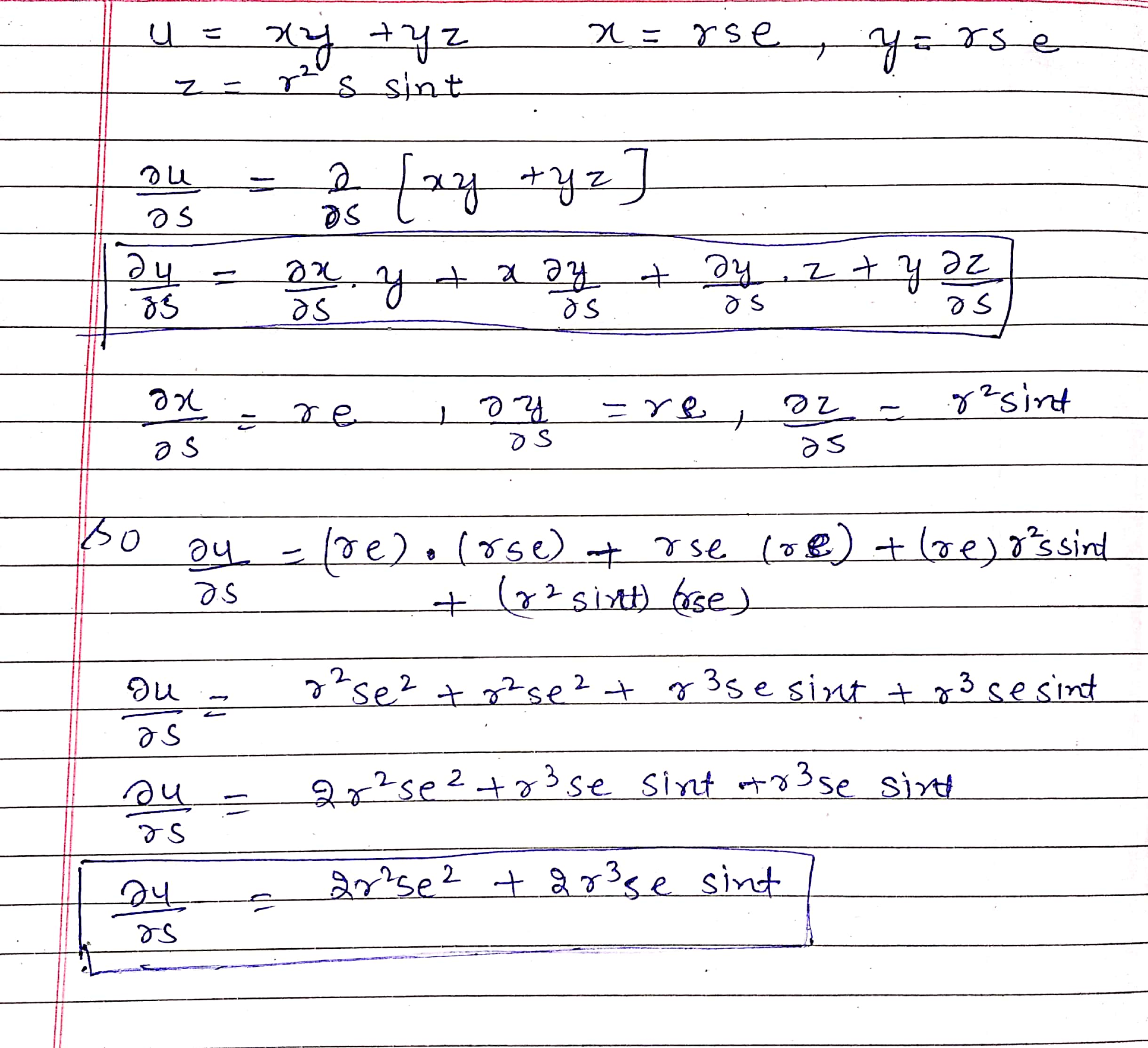#### Earn Coins

Coins can be redeemed for fabulous gifts.

Similar Homework Help Questions
• ### Use the Chain Rule to find dz/dt. (Enter your answer only in terms of t.) z...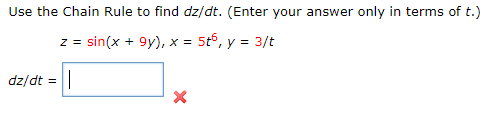Use the Chain Rule to find dz/dt. (Enter your answer only in terms of t.) z = sin(x + 9y), x = 5t6, y = 3/t dz/dt = ? Use the Chain Rule to find dz/dt. (Enter your answer only in terms of t.) z = sin(x + 9), x = 5t6, y = 3/t dz/dt =

• ### a. Use the Chain Rule to find the indicated partial derivatives. z = x4 + x2y,    x...

a. Use the Chain Rule to find the indicated partial derivatives. z = x4 + x2y,    x = s + 2t − u,    y = stu2; ∂z ∂s ∂z ∂t ∂z ∂u when s = 1, t = 2, u = 3 b. Use the Chain Rule to find the indicated partial derivatives. w = xy + yz + zx,    x = r cos(θ),    y = r sin(θ),    z = rθ; ∂w ∂r ∂w ∂θ     when r = 8, θ = pi/2 c. Use the...

• ### Chain Rule

If z=e^x*sin(y), where x=st^2 and y=s^2t, find dz/ds and dz/dt in terms of s and t using the chain rule.Thankyou!

• ### 1. +0/1 points | Previous Answers TaalmanCalc1 12.5.021 Use the Chain Rule to find the indicated ...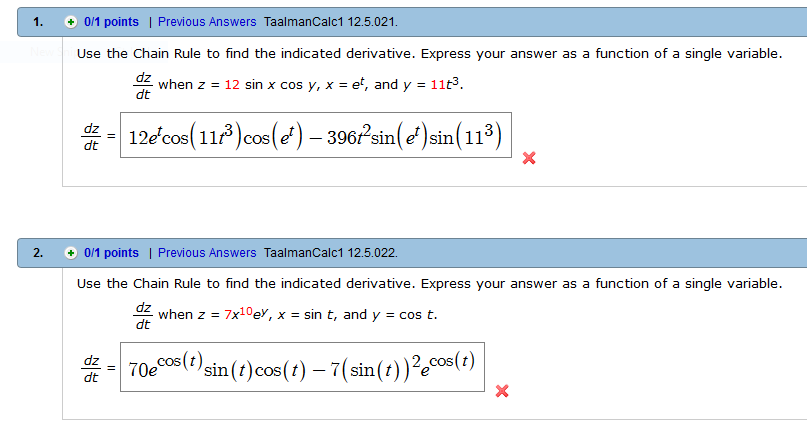1. +0/1 points | Previous Answers TaalmanCalc1 12.5.021 Use the Chain Rule to find the indicated derivative. Express your answer as a function of a single variable d when z 12 sin x cos y, x -e, and y - 113 d 12e c 11s396?sin(^sin(113) 2. 0/1 points | Previous Answers TaalmanCalc1 12.5.022. Use the Chain Rule to find the indicated derivative. Express your answer as a function of a single variable dz when z = 7x10ey, x = sin...

• ### Use the Chain Rule to find the first derivative , where z = -, x =p+q,...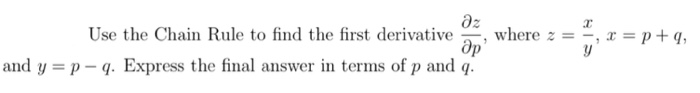Use the Chain Rule to find the first derivative , where z = -, x =p+q, and y =p - . Express the final answer in terms of p and q.

• ### (b). Use the chain rule to find and so, where w = x2 + y2 +...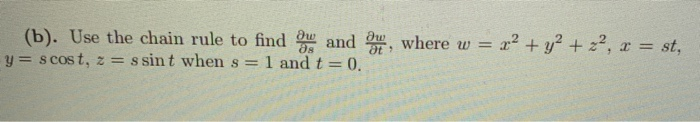(b). Use the chain rule to find and so, where w = x2 + y2 + z2, x = st, y = scost, z = s sint when s= 1 and t=0.

• ### (b). Use the chain rule to find me and where w = 22 + y2 +...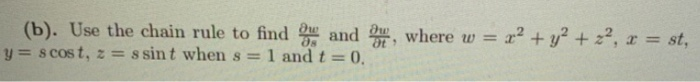(b). Use the chain rule to find me and where w = 22 + y2 + 22, x = st, y = scost, z = ssint when s = 1 and t = 0.

• ### Use the Chain Rule to find dz/dt. z = sin(x) cos(y), x= VE, y = 7/t...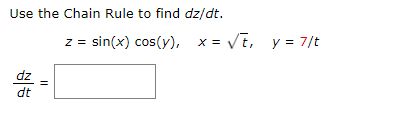Use the Chain Rule to find dz/dt. z = sin(x) cos(y), x= VE, y = 7/t dz dt 11

• ### 3. (a) Find the partial derivatives (with respect to r and s) using the chain rule:[express...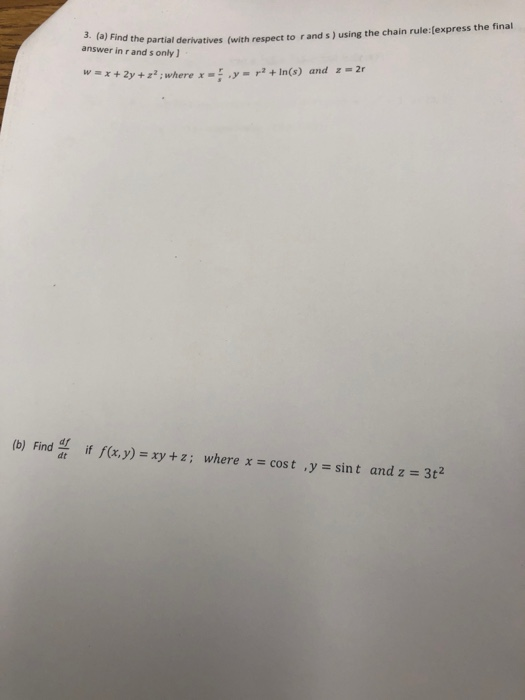3. (a) Find the partial derivatives (with respect to r and s) using the chain rule:[express the final answer in r and s only ,y= r2 +In(s) and z-2r wx2y +z2 ; where x (b) Find dt if f (x, y) = xy + z; where x cos t ,y = sint and z = 3t2

• ### Du (b). Use the chain rule to find and y = scost, z = s sint...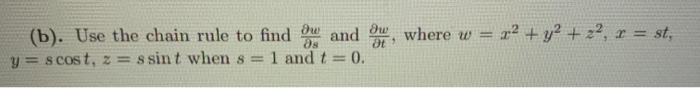Du (b). Use the chain rule to find and y = scost, z = s sint when s = 1 and t=0 Ow at where w= = 22 + y2 + 22, r = st,